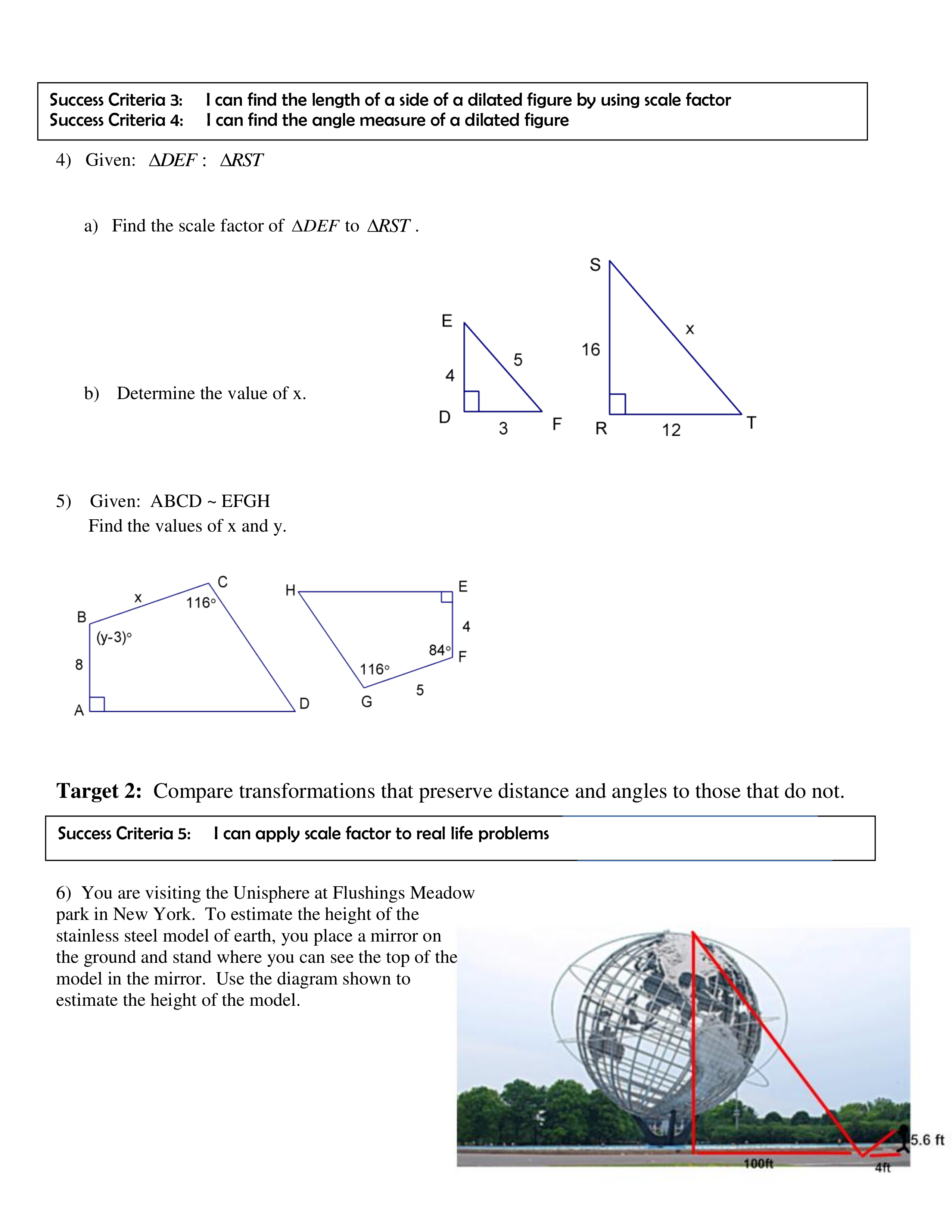Kindergarten
1st
2nd
3rd
4th
5th
6th
7th
8th
9th
10th
11th
12th
Higher Ed
Other
Subjects
ELA
Math
Science
Social Studies
Art
Computer Science
French
German
Music
Physical Education
Spanish
Other
Private Library
Final Review Sem 2 Topic 12
starstarstarstarstarstarstarstarstarstar
by Christine Bartz
| 29 Questions
Note from the author:
geometry
zoom in1
1 pt
Choose the correct statement(s)
If two polygons are similar then the corresponding sides are equal and the corresponding angles are proportional
If two polygons are similar then the corresponding sides are equal and the corresponding angles are equal
If two polygons are similar then the corresponding sides are proportional and the corresponding angles are proportional
If two polygons are similar then the corresponding sides are proportional and the corresponding angles are congruent
2
1 pt
Question 2a
3
1 pt
Question 2b
4
1 pt
Question 2c
5
1 pt
Question 2d
6
1 pt
Question 3

zoom in7
1 pt
Question 4a
8
1 pt
Question 4b
9
1 pt
Question 5. Solve for y
10
1 pt
Question 5. Solve x
11
1 pt
Question 6
zoom in12
1 pt
7a

0<|s|<1
|s|>1
s<0
|s|=1

13
1 pt
Question 7c
0<|s|<1
|s|>1
s<0
|s|=1
14
1 pt
Question 7b
0<|s|<1
|s|>1
s<0
|s|=1
15
1 pt
Question 7d
0<|s|<1
|s|>1
s<0
|s|=1
16
1 pt
Question 8
17
1 pt
Question 9
18
1 pt
Question 10
zoom in19
1 pt
Question 11
20
1 pt
Question 12.
Find the ordered pair for K'
21
1 pt
Question 12.
Find the ordered pair for L'
22
1 pt
Question 12.
Find the ordered pair for M'
23
1 pt
Question 12.
Find the ordered pair for N'
zoom in24
1 pt
Question 13
yes - True
No - False
True
False
25
1 pt
13 - Explain
AA
SSS
SAS
Sides are not proportionl
Angles are not congruent
26
1 pt
Question 14
yes - True
No - False
True
False
27
1 pt
14 - Explain
AA
SSS
SAS
Sides are not proportional
Angles are not current
28
1 pt
Question 15
yes - True
No - False
True
False
29
1 pt
Question 15 - Explain
AA
SSS
SAS
Sides are not proportional
Angles are not congruent
Add to my formatives list